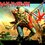# Video on the types of infinityimg

Hi!I would ask you to check out Mathemusician ViHart's latest video on the types of infinity. Click here for the video.

Here is the video description and list of topics covered from her personal blog.

$\textbf{Types of infinite numbers and some things they apply to:}$

Cardinals (set theory, applies to sizes of ordinals, sizes of Hilbert Spaces) Ordinals (set theory, used to create ordinal spaces, and in ordinal analysis. Noncommutative.) Beth Numbers (like Cardinals, or not, depending on continuum hypothesis stuff) Hyperreals (includes infinitesimals, good for analysis, computational geometry) Superreals (maximal hyperreals, similar to surreals) Supernaturals (prime factorization matters, used in field theory) Surreals (Best and most beautiful thing ever, maximal number system, combinatorial game theory) Surcomplex (surreal version of complex numbers) Infinity of Calculus (takes things to limits) Infinity of Projective Geometry (1/0=infinity, positive infinity equals negative infinity) Infinite Hilbert Space (can be any Cardinal number of dimensions) Real Line (an infinite line made up of all real numbers) Long Line (longer than the real line, in topology) Absolute infinity (self-contradictory, not really a thing)

$\textbf{Non-infinite kinds of numbers:}$

P-adic (alternative to real numbers)

Natural numbers (1, 2, 3…) Integers (…-3, -2, -1, 0, 1, 2…) Rationals (1, 1/2, 2/1, 2/3, 3/2, 3/4, 4/3…) Algebraic (sqrt 2, golden ratio, anything you can get with algebra) Transcendental (real numbers you can’t get using any finite amount of algebra, like pi and e) Reals (all possible infinite sequences of digits $0.123456789101112131415…$, includes all of the above) Imaginary (reals times i, where $i^{2}=-1$ Complex (one part real, one part “imaginary,” a consistent, commutative, associative, 2-dimensional number system) Dual numbers (instead of imagining a number where $i^2=-1$, make up a number where $ε^2=0$ and use that) Quaternions (make up numbers that square to -1, but are different from each other. $i^2=j^2=k^2=ijk=-1$. 4d, noncommutative.) Octonions (make up even more numbers, 8d, noncommutative and nonassociative.) Split-complex (imagine if $i^2=+1$, but i isn’t 1) Split-quaternions Split-octonions Bicomplex number, or tessarine Hypercomplex (category that describes/includes all complexy number systems that extend the reals)

Also see combinatorial game theory, which extends the surreal numbers to get numberlike but not-quite-number values such as “star.” Star gets confused with zero, in a mathematical definition of confusion, but it is not actually zero.

You can also write real numbers in other bases, including negative bases, irrational bases, and even complex bases.Note by Eddie The Head
7 years ago

This discussion board is a place to discuss our Daily Challenges and the math and science related to those challenges. Explanations are more than just a solution — they should explain the steps and thinking strategies that you used to obtain the solution. Comments should further the discussion of math and science.

When posting on Brilliant:

• Use the emojis to react to an explanation, whether you're congratulating a job well done , or just really confused .
• Ask specific questions about the challenge or the steps in somebody's explanation. Well-posed questions can add a lot to the discussion, but posting "I don't understand!" doesn't help anyone.
• Try to contribute something new to the discussion, whether it is an extension, generalization or other idea related to the challenge.
• Stay on topic — we're all here to learn more about math and science, not to hear about your favorite get-rich-quick scheme or current world events.

MarkdownAppears as
*italics* or _italics_ italics
**bold** or __bold__ bold
- bulleted- list
• bulleted
• list
1. numbered2. list
1. numbered
2. list
Note: you must add a full line of space before and after lists for them to show up correctly
paragraph 1paragraph 2

paragraph 1

paragraph 2

[example link](https://brilliant.org)example link
> This is a quote
This is a quote
    # I indented these lines
# 4 spaces, and now they show
# up as a code block.

print "hello world"
# I indented these lines
# 4 spaces, and now they show
# up as a code block.

print "hello world"
MathAppears as
Remember to wrap math in $$ ... $$ or $ ... $ to ensure proper formatting.
2 \times 3 $2 \times 3$
2^{34} $2^{34}$
a_{i-1} $a_{i-1}$
\frac{2}{3} $\frac{2}{3}$
\sqrt{2} $\sqrt{2}$
\sum_{i=1}^3 $\sum_{i=1}^3$
\sin \theta $\sin \theta$
\boxed{123} $\boxed{123}$

## Comments

There are no comments in this discussion.

×

Problem Loading...

Note Loading...

Set Loading...